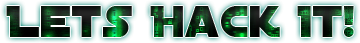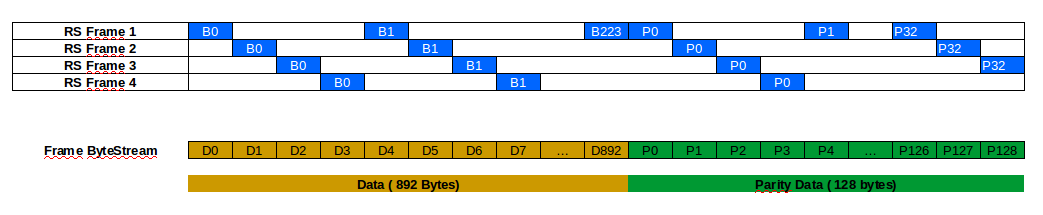# Reed Solomon Error Correction

Other of the things that were missing on the last part is the Data Error Correction. We already did the Foward Error Correction (FEC, the viterbi), but we also can do Reed Solomon. Notice that Reed Solomon is completely optional if you have good SNR (that is better than 9dB and viterbi less than 50 BER) since ReedSolomon doesn’t alter the data. I prefer to use RS because I don’t have a perfect signal (although my average RS corrections are 0) and I want my packet data to be consistent. The RS doesn’t usually add to much overhead, so its not big deal to use. Also the libfec provides a RS algorithm for the CCSDS standard.

I will assume you have a uint8_t buffer with a frame data of 1020 bytes (that is, the data we got in the last chapter with the sync word excluded). The CCSDS standard RS uses 255,223 as the parameters. That means that each RS Frame has 255 bytes which 223 bytes are data and 32 bytes are parity. With this specs, we can correct any 16 bytes in our 223 byte of data. In our LRIT Frame we have 4 RS Frames, but the structure are not linear. Since the Viterbi uses a Trellis diagram, the error in Trellis path can generate a sequence of bad bytes in the stream. So if we had a linear sequence of RS Frames, we could corrupt a lot of bytes from one frame and lose one of the RS Frames (that means that we lose the entire LRIT frame). So the data is interleaved by byte. The image below shows how the data is spread over the lrit frame.For correcting the data, we need to de-interleave to generate the four RS Frames, run the RS algorithm and then interleave again to have the frame data. The [de]interleaving process are very simple. You can use these functions to do that:

``````#define PARITY_OFFSET 892
void deinterleaveRS(uint8_t *data, uint8_t *rsbuff, uint8_t pos, uint8_t I) {
// Copy data
for (int i=0; i<223; i++) {
rsbuff[i] = data[i*I + pos];
}
// Copy parity
for (int i=0; i<32; i++) {
rsbuff[i+223] = data[PARITY_OFFSET + i*I + pos];
}
}

void interleaveRS(uint8_t *idata, uint8_t *outbuff, uint8_t pos, uint8_t I) {
// Copy data
for (int i=0; i<223; i++) {
outbuff[i*I + pos] = idata[i];
}
// Copy parity - Not needed here, but I do.
for (int i=0; i<32; i++) {
outbuff[PARITY_OFFSET + i*I + pos] = idata[i+223];
}
}
``````

For using it on LRIT frame we can do:

``````#define RSBLOCKS 4
int derrors = { 0, 0, 0, 0 };
uint8_t rsWorkBuffer;
uint8_t rsCorrectedData;

for (int i=0; i<RSBLOCKS; i++) {
deinterleaveRS(decodedData, rsWorkBuffer, i, RSBLOCKS);
derrors[i] = decode_rs_ccsds(rsWorkBuffer, NULL, 0, 0);
interleaveRS(rsWorkBuffer, rsCorrectedData, i, RSBLOCKS);
}
``````

In the variable derrors we will have how many bytes it was corrected for each RS Frames. In rsCorrectedData we will have the error corrected output. The value -1 in derrors it means the data is corrupted beyond correction (or the parity is corrupted beyond correction). I usually drop the entire frame if all derrors are -1, but keep in mind that the corruption can happen in the parity only (we can have corrupted bytes in parity that will lead to -1 in error correction) so it would be wise to not do like I did. After that we will have the corrected LRIT Frame that is 892 bytes wide.